Home >  > GBDT + LR预测股价

## GBDT + LR预测股价

0

LR属于线性模型，容易并行化，可以轻松处理上亿条数据，但是学习能力十分有限，需要大量的特征工程来增加模型的学习能力。但大量的特征工程耗时耗力同时并不一定会带来效果提升。因此，如何自动发现有效的特征、特征组合，弥补人工经验不足，缩短LR特征实验周期，是亟需解决的问题。

1）为什么要使用集成的决策树模型，而不是单棵的决策树模型：一棵树的表达能力很弱，不足以表达多个有区分性的特征组合，多棵树的表达能力更强一些。可以更好的发现有效的特征和特征组合
2）为什么建树采用GBDT而非RF：RF也是多棵树，但从效果上有实践证明不如GBDT。且GBDT前面的树，特征分裂主要体现对多数样本有区分度的特征；后面的树，主要体现的是经过前N颗树，残差仍然较大的少数样本。优先选用在整体上有区分度的特征，再选用针对少数样本有区分度的特征，思路更加合理，这应该也是用GBDT的原因。

1.Normalized Cross-Entropy(NE)
2.lgb.Dataset 使用Dataset构建数据到lgb中。

```import lightgbm as lgb
import numpy as np

# 训练数据，500个样本，10个维度
train_data = np.random.rand(500, 10)
# 构建二分类数据
label = np.random.randint(2, size=500)
# 放入到dataset中
train = lgb.Dataset(train_data, label=label)

train_data = lgb.Dataset(data, label=label, feature_name=['c1', 'c2', 'c3'], categorical_feature=['c3'])
```

3.lgb_eval 创建验证数据

4.lgb.train
lgb参数的参数可以看这里：https://blog.csdn.net/qq_23069955/article/details/80611701
LGB(机器学习模板)：https://blog.csdn.net/sunshunli/article/details/82830969

XGBoost Plotting API以及GBDT组合特征实践：https://blog.csdn.net/zhangf666/article/details/70183788

5.num_trees
print(np.array(y_pred).shape)
print(y_pred[:1])的输出结果：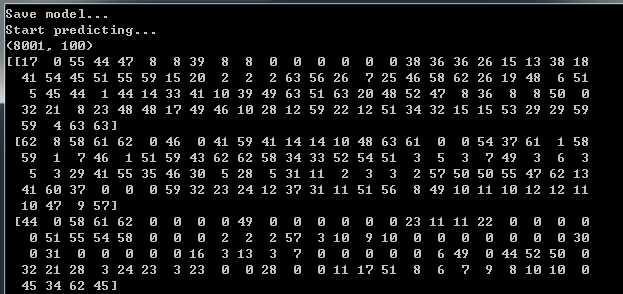6.temp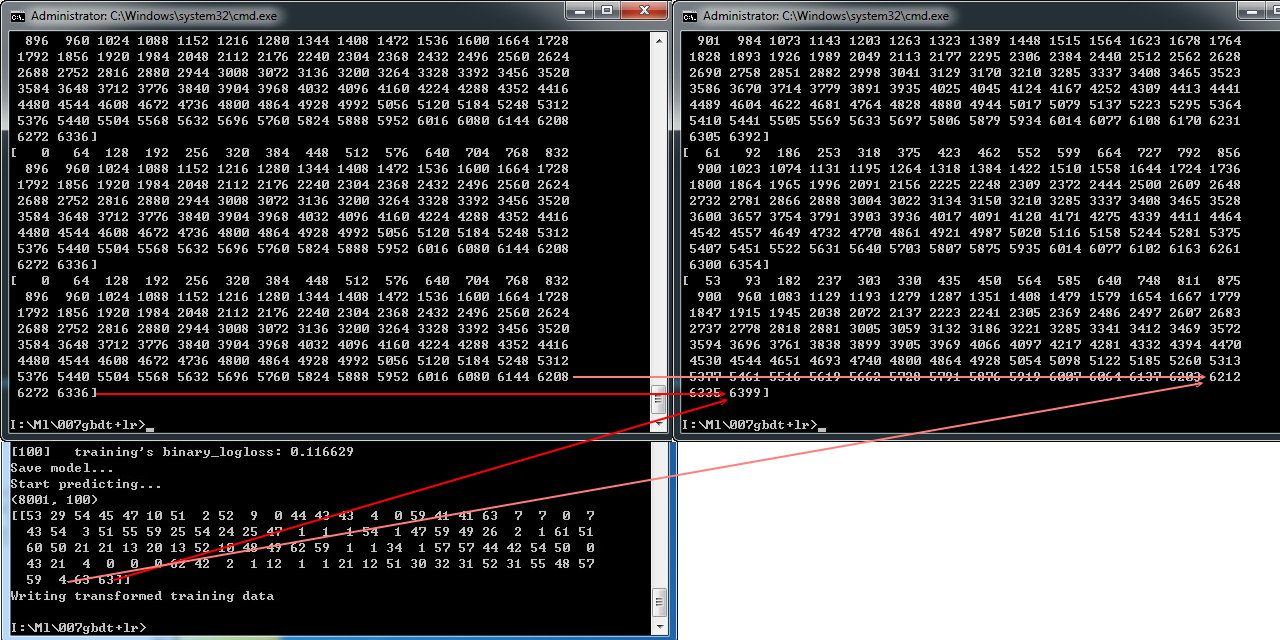https://lightgbm.apachecn.org/#/

7.lm.predict_proba

8.lm = LogisticRegression(penalty='l2',C=0.05)
penalty：惩罚项，str类型，可选参数为l1和l2，默认为l2。用于指定惩罚项中使用的规范。newton-cg、sag和lbfgs求解算法只支持L2规范。L1G规范假设的是模型的参数满足拉普拉斯分布，L2假设的模型参数满足高斯分布，所谓的范式就是加上对参数的约束，使得模型更不会过拟合(overfit)，但是如果要说是不是加了约束就会好，这个没有人能回答，只能说，加约束的情况下，理论上应该可以获得泛化能力更强的结果。

c：正则化系数λ的倒数，float类型，默认为1.0。必须是正浮点型数。像SVM一样，越小的数值表示越强的正则化。

9.transformed_training_matrix[i][temp]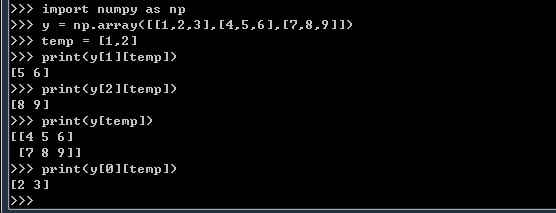```print(transformed_training_matrix)  #结果为(8001, 6400)
for i in range(0, len(y_pred)):
temp = np.arange(len(y_pred)) * num_leaf + np.array(y_pred[i])
print(temp)
transformed_training_matrix[i][temp] += 1  #意思是将temp中数据对应的列的值加上1
print(len(transformed_training_matrix[i]))

```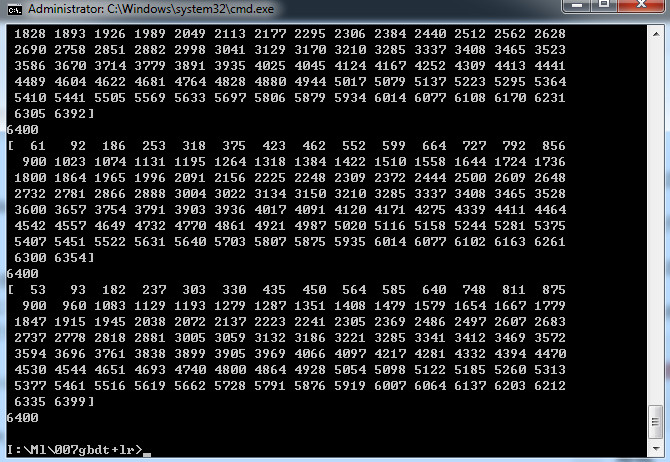3.面板回归与截面回归

4.对数据的假设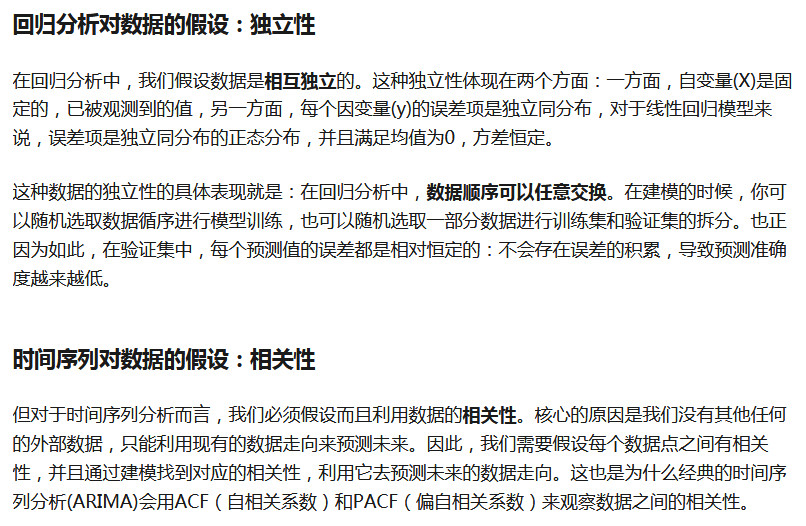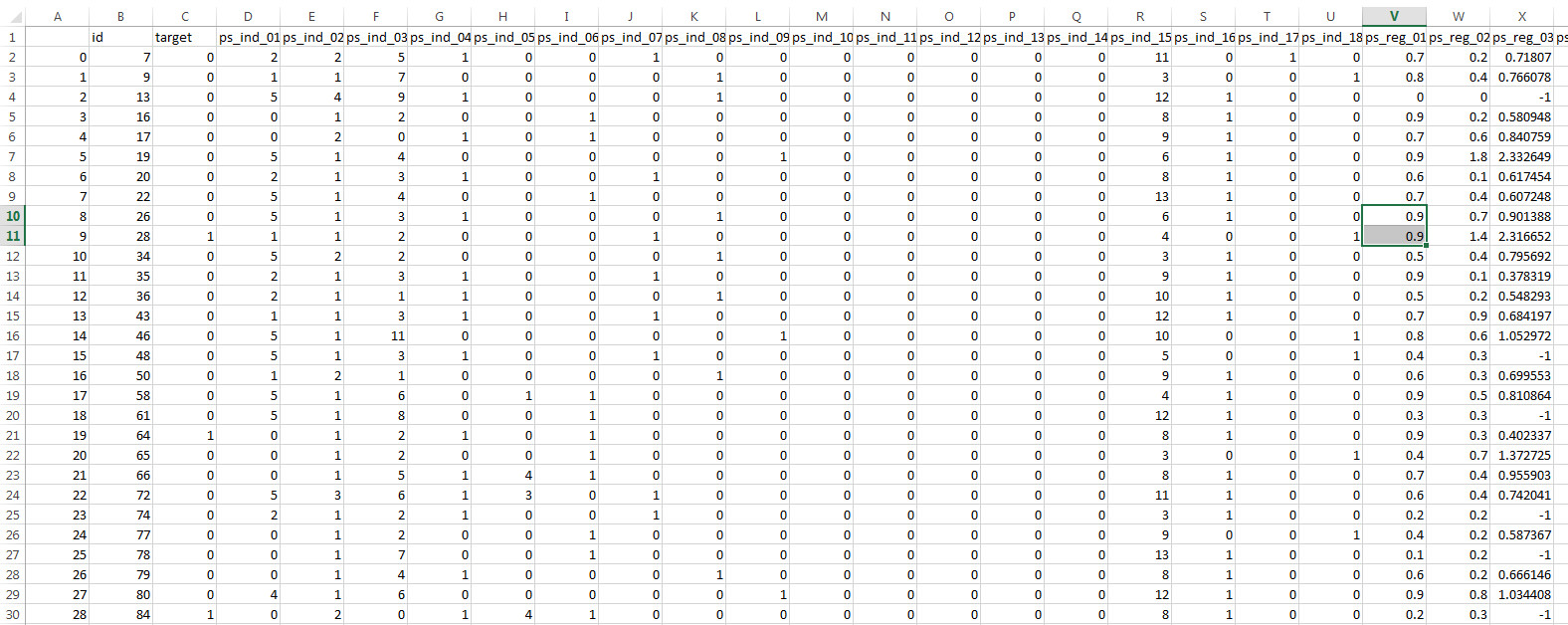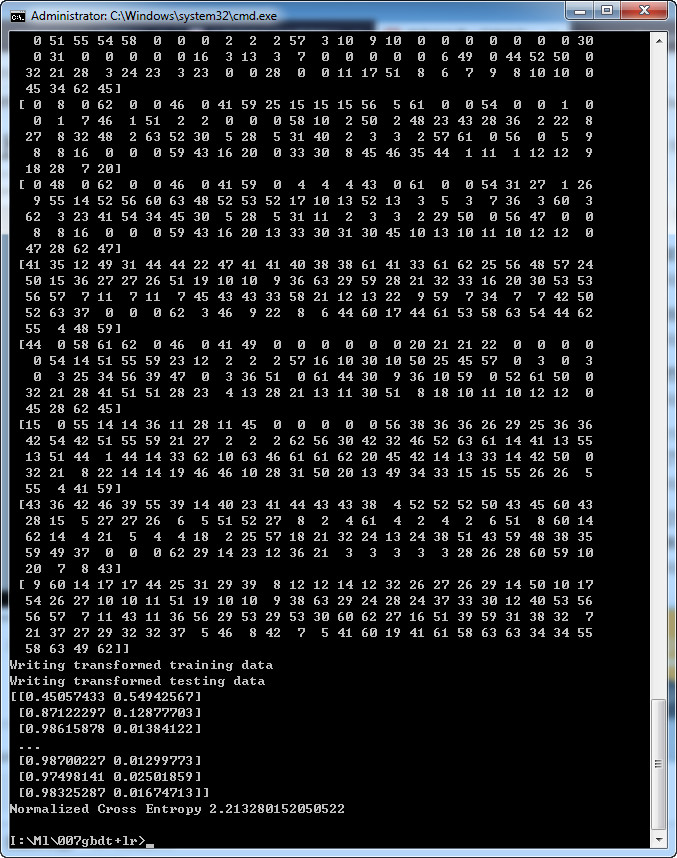model.txt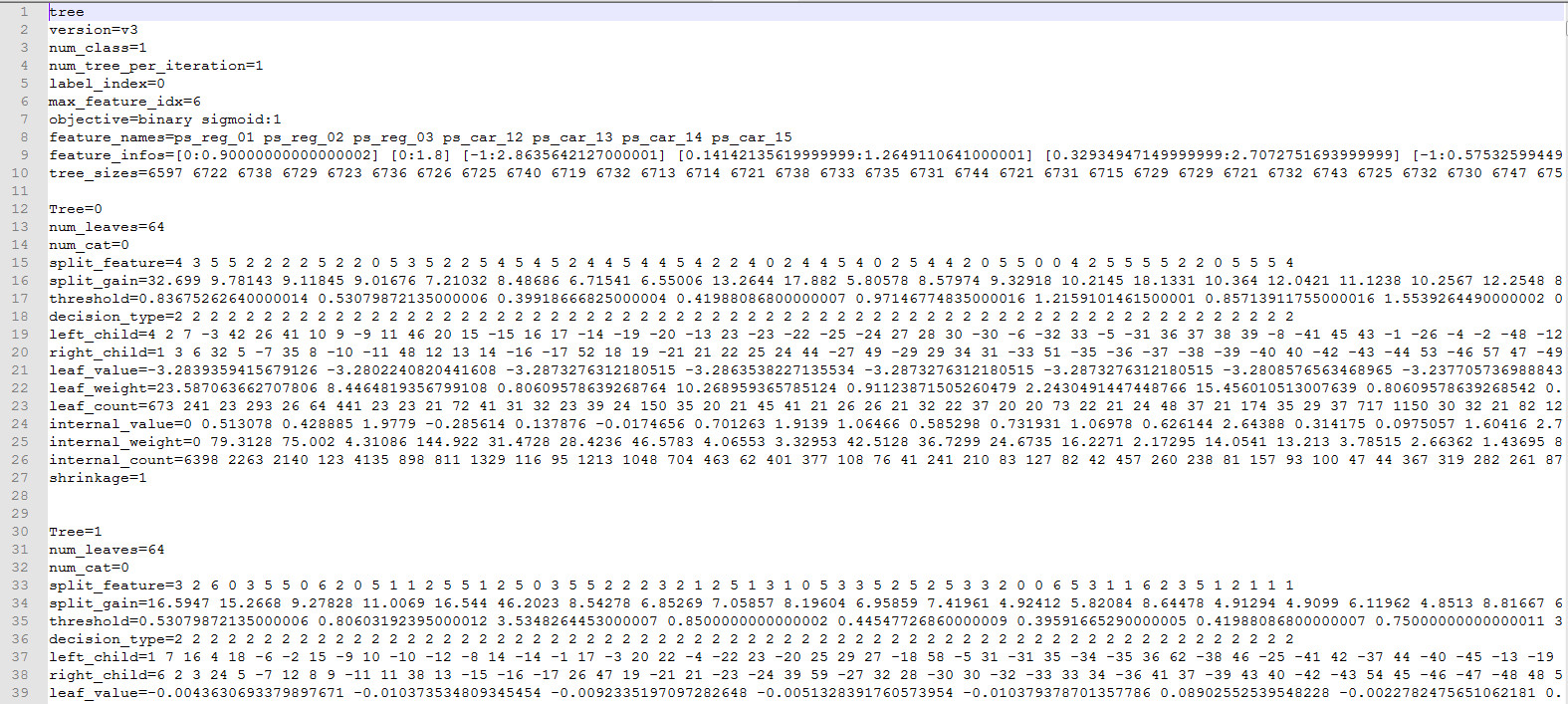```import lightgbm as lgb

import pandas as pd
import numpy as np

from sklearn.metrics import mean_squared_error
from sklearn.linear_model import LogisticRegression

NUMERIC_COLS = [
"ps_reg_01", "ps_reg_02", "ps_reg_03",
"ps_car_12", "ps_car_13", "ps_car_14", "ps_car_15",
]

y_train = df_train['target']  # training label
y_test = df_test['target']  # testing label
X_train = df_train[NUMERIC_COLS]  # training dataset
X_test = df_test[NUMERIC_COLS]  # testing dataset

# create dataset for lightgbm
lgb_train = lgb.Dataset(X_train, y_train)
lgb_eval = lgb.Dataset(X_test, y_test, reference=lgb_train)

params = {
'boosting_type': 'gbdt',
'objective': 'binary',         # 比如要完成预测用户是否买单行为，所以是binary，不买是0，购买是1
'metric': {'binary_logloss'},  # 评判指标
'num_leaves': 64,              # 大会更准,但可能过拟合
'num_trees': 100,
'learning_rate': 0.01,         # 学习率
'feature_fraction': 0.9,       # 防止过拟合
'bagging_fraction': 0.8,       # 防止过拟合
'bagging_freq': 5,             # 防止过拟合
'verbose': 0
}

# number of leaves,will be used in feature transformation
num_leaf = 64

print('Start training...')
# train
gbm = lgb.train(params,
lgb_train,
num_boost_round=100,
valid_sets=lgb_train)

print('Save model...')
# save model to file
gbm.save_model('model.txt')

print('Start predicting...')
# predict and get data on leaves, training data
#　当设置pred_leaf=True的时候, 这时就会输出每个样本在所有树中的叶子节点。
y_pred = gbm.predict(X_train, pred_leaf=True)

print(np.array(y_pred).shape)　　　＃输出结果为(8001, 100)这样的形式
print(y_pred[:10])

print('Writing transformed training data')
transformed_training_matrix = np.zeros([len(y_pred), len(y_pred) * num_leaf],
dtype=np.int64)  # N * num_tress * num_leafs，生成一个8001，100*64的np
#print(len(y_pred))           #结果为8100
#print(len(y_pred))        #结果为100
#print(transformed_training_matrix.shape)   #结果为(8001, 6400)
for i in range(0, len(y_pred)):
temp = np.arange(len(y_pred)) * num_leaf + np.array(y_pred[i])  ##这段代码的意思是生成一个1-100的列表*64+每个样本在所有树中的叶子节点
transformed_training_matrix[i][temp] += 1

y_pred = gbm.predict(X_test, pred_leaf=True)
print('Writing transformed testing data')
transformed_testing_matrix = np.zeros([len(y_pred), len(y_pred
lm = LogisticRegression(penalty='l2',C=0.05) # logestic model construction
lm.fit(transformed_training_matrix,y_train)  # fitting the data
y_pred_test = lm.predict_proba(transformed_testing_matrix)   # Give the probabilty on each label

print(y_pred_test)

NE = (-1) / len(y_pred_test) * sum(((1+y_test)/2 * np.log(y_pred_test[:,1]) +  (1-y_test)/2 * np.log(1 - y_pred_test[:,1])))
print("Normalized Cross Entropy " + str(NE))
```

1.https://zhuanlan.zhihu.com/p/37522339
2.https://www.toutiao.com/i6644852565341110791/